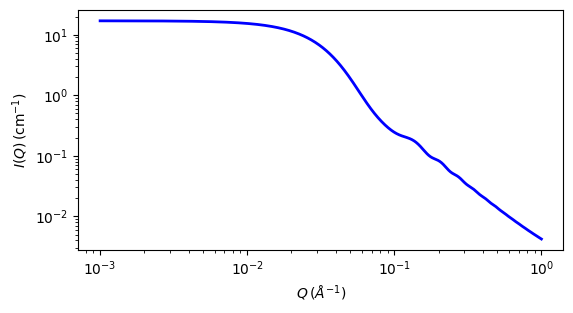# polymer_micelle

Polymer micelle model

Parameter

Description

Units

Default value

scale

Scale factor or Volume fraction

None

1

background

Source background

cm-1

0.001

ndensity

Number density of micelles

1015cm3

8.94

v_core

Core volume

3

62624

v_corona

Corona volume

3

61940

sld_solvent

Solvent scattering length density

10-6-2

6.4

sld_core

Core scattering length density

10-6-2

0.34

sld_corona

Corona scattering length density

10-6-2

0.8

Radius of core ( must be >> rg )

45

rg

Radius of gyration of chains in corona

20

d_penetration

Factor to mimic non-penetration of Gaussian chains

None

1

n_aggreg

Aggregation number of the micelle

None

6

The returned value is scaled to units of cm-1 sr-1, absolute scale.

This model provides the form factor, $$P(q)$$, for a micelle with a spherical core and Gaussian polymer chains attached to the surface, thus may be applied to block copolymer micelles. To work well the Gaussian chains must be much smaller than the core, which is often not the case. Please study the reference carefully.

Definition

The 1D scattering intensity for this model is calculated according to the equations given by Pedersen (Pedersen, 2000), summarised briefly here.

The micelle core is imagined as $$N$$ = n_aggreg polymer heads, each of volume $$V_\text{core}$$, which then defines a micelle core of radius $$r$$ = r_core, which is a separate parameter even though it could be directly determined. The Gaussian random coil tails, of gyration radius $$R_g$$, are imagined uniformly distributed around the spherical core, centred at a distance $$r + d \cdot R_g$$ from the micelle centre, where $$d$$ = d_penetration is of order unity. A volume $$V_\text{corona}$$ is defined for each coil. The model in detail seems to separately parameterize the terms for the shape of $$I(Q)$$ and the relative intensity of each term, so use with caution and check parameters for consistency. The spherical core is monodisperse, so it’s intensity and the cross terms may have sharp oscillations (use $$q$$ resolution smearing if needs be to help remove them).

$\begin{split}P(q) &= N^2\beta^2_s\Phi(qr)^2 + N\beta^2_cP_c(q) + 2N^2\beta_s\beta_cS_{sc}(q) + N(N-1)\beta_c^2S_{cc}(q) \\ \beta_s &= V_\text{core}(\rho_\text{core} - \rho_\text{solvent}) \\ \beta_c &= V_\text{corona}(\rho_\text{corona} - \rho_\text{solvent})\end{split}$

where $$\rho_\text{core}$$, $$\rho_\text{corona}$$ and $$\rho_\text{solvent}$$ are the scattering length densities sld_core, sld_corona and sld_solvent. For the spherical core of radius $$r$$

$\Phi(qr)= \frac{\sin(qr) - qr\cos(qr)}{(qr)^3}$

whilst for the Gaussian coils

$\begin{split}P_c(q) &= 2 [\exp(-Z) + Z - 1] / Z^2 \\ Z &= (q R_g)^2\end{split}$

The sphere to coil (core to corona) and coil to coil (corona to corona) cross terms are approximated by:

$\begin{split}S_{sc}(q) &= \Phi(qr)\psi(Z) \frac{\sin(q(r+d \cdot R_g))}{q(r+d \cdot R_g)} \\ S_{cc}(q) &= \psi(Z)^2 \left[\frac{\sin(q(r+d \cdot R_g))}{q(r+d \cdot R_g)} \right]^2 \\ \psi(Z) &= \frac{[1-\exp^{-Z}]}{Z}\end{split}$

Validation

$$P(q)$$ above is multiplied by ndensity, and a units conversion of $$10^{-13}$$, so scale is likely 1.0 if the scattering data is in absolute units. This model has not yet been independently validated.Fig. 86 1D plot corresponding to the default parameters of the model.

Source

polymer_micelle.py $$\ \star\$$ polymer_micelle.c $$\ \star\$$ sas_3j1x_x.c

References

1. J Pedersen, J. Appl. Cryst., 33 (2000) 637-640

Authorship and Verification

• Translated by : Richard Heenan Date: March 20, 2016